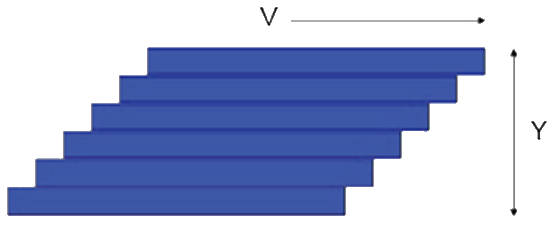## Viscosity Basics

### Quick Start

The only problem with viscosity is with the meanings of shear, stress and shear strain rate (or shear rate). Here they are all explained and the most basic calculations done for you.

### Viscosity Basics

V m/s
Y mm
γ̇ /s
η Pa.s
γ̇ /s
τ PaThe starting point is a pack of cards of height Y and with a force σ moving the top card with a velocity V. There is friction between the cards and the card on the bottom is immovable. For a given inter-card friction, the force σ will need to double if V is doubled for obvious reasons. It also has to be doubled if Y is halved because the velocity gradient, V/Y is much steeper so each card feels more of the influence of the fixed card. If the inter-card friction is called η then

σ=(ηV)/Y

Now substitute "streamline" for card and we have the equation for the force required to push the surface of a liquid of thickness Y at velocity V. V/Y has units of (m/s)/m = 1/s and is the "shear strain rate" which is given the symbol γ̇. So:

σ=ηγ̇

Rheologists tend to call γ̇ "strain rate" and the rest of us call it "shear rate" or just "shear". Because shear rate is in s-1 and shear stress is in Pa (i.e. force/area = N/m2) then viscosity, η=σ/(γ̇), is in Pa.s.